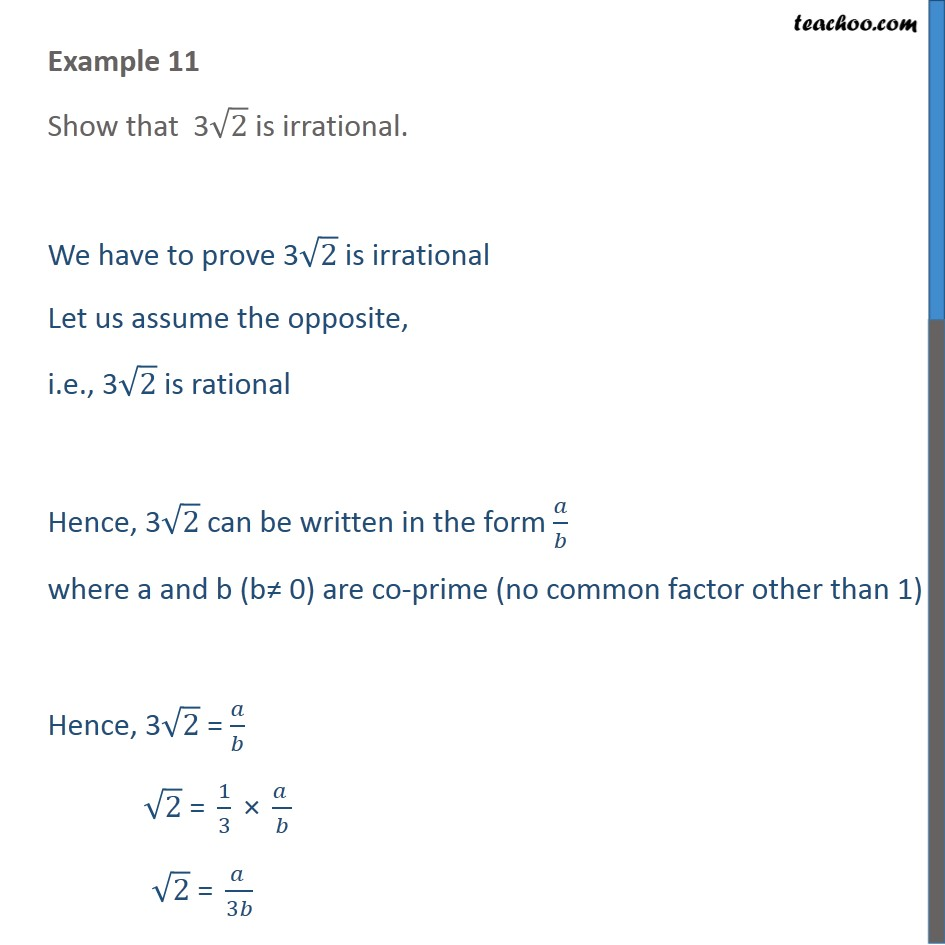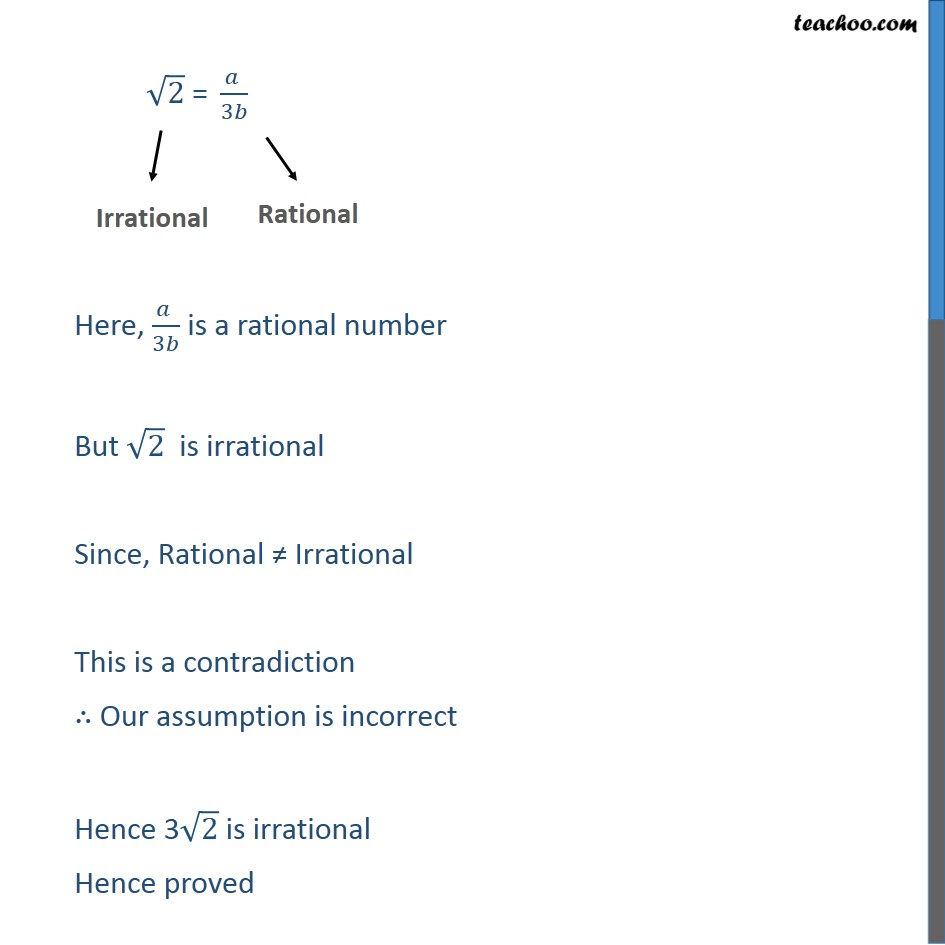1. Chapter 1 Class 10 Real Numbers
2. Concept wise
3. Irrational numbers

Transcript

Example 11 Show that 3√2 is irrational. We have to prove 3√2 is irrational Let us assume the opposite, i.e., 3√2 is rational Hence, 3√2 can be written in the form 𝑎/𝑏 where a and b (b≠ 0) are co-prime (no common factor other than 1) Hence, 3√2 = 𝑎/𝑏 √2 " = " 1/3 " × " (𝑎 )/𝑏 " " √2 " = " (𝑎 )/3𝑏 √2 " = " (𝑎 )/3𝑏 Here, (𝑎 )/3𝑏 is a rational number But √2 is irrational Since, Rational ≠ Irrational This is a contradiction ∴ Our assumption is incorrect Hence 3√2 is irrational Hence proved

Irrational numbers